# Scenario Generation from Recorded Vehicle Data

This example shows how to generate a virtual driving scenario from recorded vehicle data. The scenario is generated from position information recorded from a GPS sensor and recorded object lists processed from a lidar sensor.

### Overview

Virtual driving scenarios can be used to recreate a real scenario from recorded vehicle data. These virtual scenarios enable you to visualize and study the original scenario. Because you can programmatically modify virtual scenarios, you can also use them to synthesize scenario variations when designing and evaluating autonomous driving systems.

In this example, you create a virtual driving scenario by generating a `drivingScenario`` `object from data that was recorded from a test (ego) vehicle and an ASAM OpenDRIVE® file. The ASAM OpenDRIVE file describes the road network of the area where the data was recorded. The recorded vehicle data includes:

• GPS data: Text file containing the latitude and longitude coordinates of the ego vehicle at each timestamp.

• Lidar object list data: Text file containing the number of non-ego actors and the positions of their centers, relative to the ego vehicle, at each timestamp.

• Video data: MP4 file recorded from a forward-facing monocular camera mounted on the ego vehicle.

To generate and simulate the driving scenario, you follow these steps:

1. Explore recorded vehicle data.

2. Import ASAM OpenDRIVE road network into driving scenario.

3. Add ego vehicle data from GPS to driving scenario.

4. Add non-ego actors from lidar object list to driving scenario.

5. Simulate and visualize generated scenario.

The following diagram shows how you use the recorded data in this example. Notice that you create the driving scenario from the GPS, lidar object lists, and ASAM OpenDRIVE files. You use the camera data to visualize the original scenario and can compare this data with the scenario you generate. You also visualize the scenario route on a map using `geoplayer`.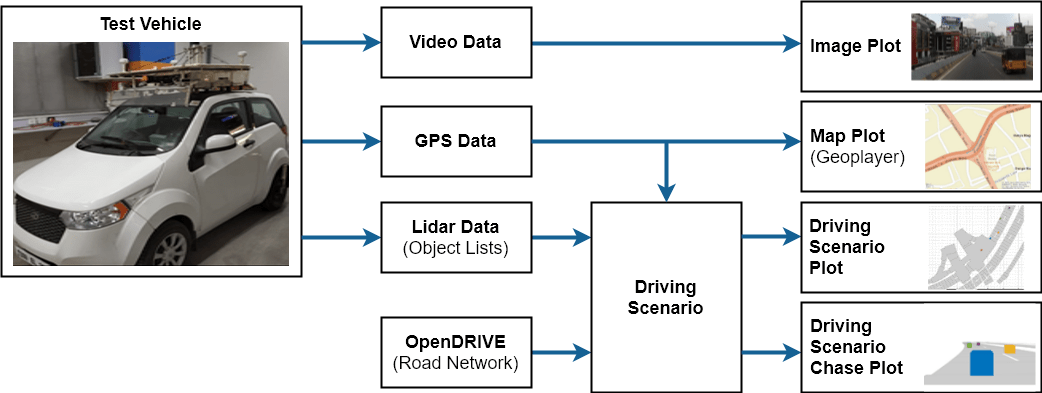### Explore Recorded Vehicle Data

The positions of the ego vehicle were captured using a UBlox GPS NEO M8N sensor. The GPS sensor was placed on the center of the roof of the ego vehicle. This data is saved in the text file `EgoUrban.txt`.

The positions of the non-ego actors were captured using a Velodyne® VLP-16 lidar sensor with a range of 30 meters. The VLP-16 sensor was placed on the roof of the ego vehicle at a position and height that avoids having the sensor collide with the body of the ego vehicle. The point cloud from the lidar sensor was processed on the vehicle to detect objects and their positions relative to the ego vehicle. This data is saved in the text file `NonEgoUrban.txt`.

To help understand the original scenario, video from a monocular camera was recorded as a reference. This video can also be used to visually compare the original and generated scenarios. A preview of this recorded video is saved in the video file `urbanpreview.mp4`. You can download the full recorded video file from here.

Generate a preview of the urban traffic scenario used in this example.

```vidObj = VideoReader("urbanpreview.mp4"); fig = figure; set(fig,Position=[0, 0, 800, 600]); movegui(fig,"center"); pnl = uipanel(fig,Position=[0 0 1 1],... Title="Urban Traffic Scenario"); plt = axes(pnl); while hasFrame(vidObj) vidFrame = readFrame(vidObj); image(vidFrame,Parent=plt); plt.Visible = "off"; pause(1/vidObj.FrameRate); end```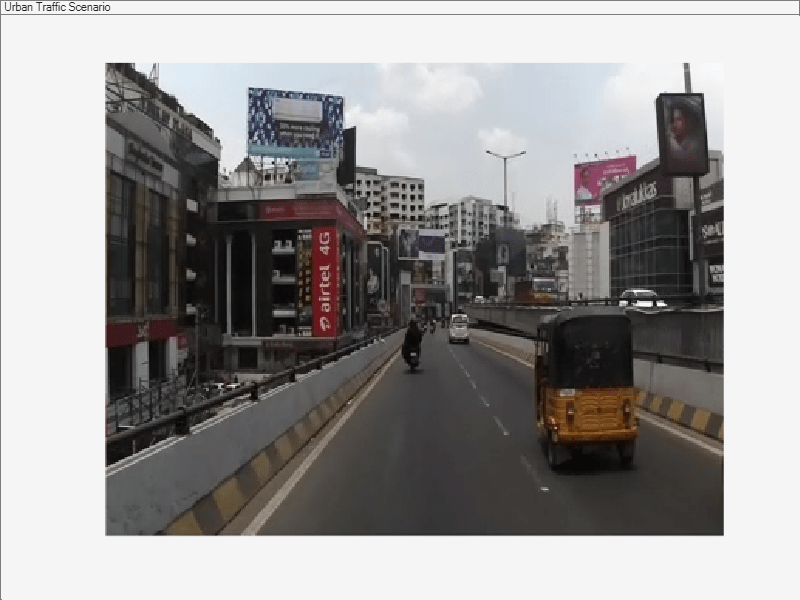Though the sensor coverage area can be defined around the entire ego vehicle, this example shows only the forward-looking scenario.

### Import ASAM OpenDRIVE Road Network into Driving Scenario

The road network data for generating the virtual scenario is obtained from OpenStreetMap®. The OpenStreetMap data files are converted to ASAM OpenDRIVE files and saved with extension `.xodr`. Use the `roadNetwork` function to import this road network data to a driving scenario.

Create a driving scenario object and import the desired ASAM OpenDRIVE road network into the generated scenario.

```scenario = drivingScenario; openDRIVEFile = "OpenDRIVEUrban.xodr"; roadNetwork(scenario,"OpenDRIVE",openDRIVEFile);```

### Add Ego Vehicle Data from GPS to Generated Scenario

The ego vehicle data is collected from the GPS sensor and stored as a text file. The text file consists of three columns that store the latitude, longitude, and timestamp values for the ego vehicle. Use the `helperGetEgoData` function to import the ego vehicle data from the text file into a structure in the MATLAB® workspace. The structure contains three fields specifying the latitude, longitude and timestamps.

```egoFile = "EgoUrban.txt"; egoData = helperGetEgoData(egoFile);```

Compute the trajectory waypoints of the ego vehicle from the recorded GPS coordinates. Use the `latlon2local` function to convert the raw GPS coordinates to the local east-north-up Cartesian coordinates. The transformed coordinates define the trajectory waypoints of the ego vehicle.

```% Specify latitude and longitude at origin of data from ASAM OpenDRIVE file. This point will also define the origin of the local coordinate system. alt = 540.0 % Average altitude in Hyderabad, India```
```alt = 540 ```
```origin = [17.425853702697903, 78.44939480188313, alt]; % [lat, lon, altitude] % Specify latitude and longitude of ego vehicle lat = egoData.lat; lon = egoData.lon; % Compute waypoints of ego vehicle [X,Y,~] = latlon2local(lat,lon,alt,origin); egoWaypoints(:,1) = X; egoWaypoints(:,2) = Y;```

Visualize the GPS path of the ego vehicle using the `geoplayer` object.

```zoomLevel = 17; player = geoplayer(lat(1),lon(1),zoomLevel); plotRoute(player,lat,lon); for i = 1:size(lat,1) plotPosition(player,lat(i),lon(i)); end```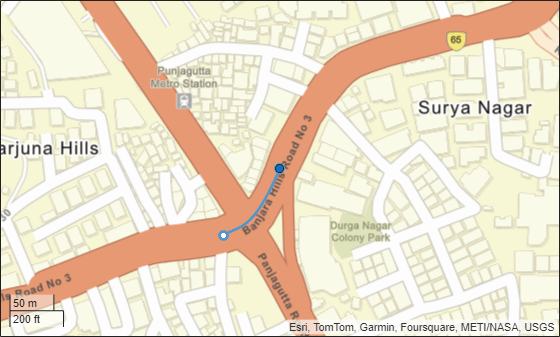Use `helperComputeEgoData` to compute the speed and the heading angle values of the ego vehicle at each sensor data timestamp.

`[egoSpeed,egoAngle] = helperComputeEgoData(egoData,X,Y);`

Add the ego vehicle to the driving scenario.

```ego = vehicle(scenario,ClassID=1,Name="Ego",... Length=3.6,Width=1.55,Height=1.6,... Mesh=driving.scenario.carMesh);```

Create a trajectory for the ego vehicle from the computed set of ego waypoints and the speed. The ego vehicle follows the trajectory at the specified speed.

`trajectory(ego,egoWaypoints,egoSpeed);`

### Add Non-Ego Actors from Lidar Object Lists to Generated Scenario

The non-ego actor data is collected from the lidar sensor and stored as a text file. The text file consists of five columns that store the actor IDs, `x`-positions, `y`-positions, `z`-positions and timestamp values, respectively. Use the `helperGetNonEgoData` function to import the non-ego actor data from the text file into a structure in the MATLAB® workspace. The output is a structure with three fields:

1. `ActorID` - Scenario-defined actor identifier, specified as a positive integer.

2. `Position - `Position of actor, specified as an [x y z] real vector. Units are in meters.

3. `Time` - Timestamp of the sensor recording.

```nonEgoPosFile = "NonEgoUrban.txt"; nonEgoPropertiesFile = "NonEgoProperties.txt"; [nonEgoData, nonEgoProperties] = ... helperGetNonEgoData(nonEgoPosFile, nonEgoPropertiesFile);```

Use `helperComputeNonEgoData` to compute the trajectory waypoints and the speed of each non-ego actor at each timestamp. The trajectory waypoints are computed relative to the ego vehicle.

```actors = unique(nonEgoData(1).ActorID); [nonEgoSpeed, nonEgoWaypoints] = ... helperComputeNonEgoData(egoData,... egoWaypoints,nonEgoData,egoAngle);```

Determine the mesh for non-ego actor according to their class ID.

```for i = 1:size(nonEgoProperties.ClassID,1) switch nonEgoProperties.ClassID(i) case 3 nonEgoProperties.Mesh(i,1) = driving.scenario.bicycleMesh; case 2 nonEgoProperties.Mesh(i,1) = driving.scenario.truckMesh; otherwise nonEgoProperties.Mesh(i,1) = driving.scenario.carMesh; end end```

Add the non-ego actors to the driving scenario.You can populate the non-ego actors with appropriate class ID, dimension, colour and mesh. Create trajectories for the non-ego actors from the computed set of actor waypoints and the speed.

```for i = 1:size(actors,1) actor = vehicle(scenario,ClassID=1,... Length=nonEgoProperties.Length(i),... Width=nonEgoProperties.Width(i),... Height=nonEgoProperties.Height(i),... PlotColor=nonEgoProperties.Color(i,:),... Mesh=nonEgoProperties.Mesh(i)); trajectory(actor,nonEgoWaypoints{i},nonEgoSpeed{i}); end```

Visualize the ego vehicle and non-ego actors that you imported into the generated scenario. Also visualize the corresponding trajectory waypoints of the ego vehicle and non-ego actors.

```% Create a custom figure window and define an axes object fig = figure; set(fig,Position=[0, 0, 800, 600]); movegui(fig,"center"); hViewPnl = uipanel(fig,Position=[0 0 1 1],... Title="Ego Vehicle and Actors"); hCarPlt = axes(hViewPnl); % Plot the generated driving scenario. plot(scenario,"Parent",hCarPlt); axis([270 320 80 120]); legend("Imported Road Network","Lanes","Ego Vehicle",... "Actor 1","Actor 2","Actor 3","Actor 4","Actor 5") legend(hCarPlt,"boxoff");```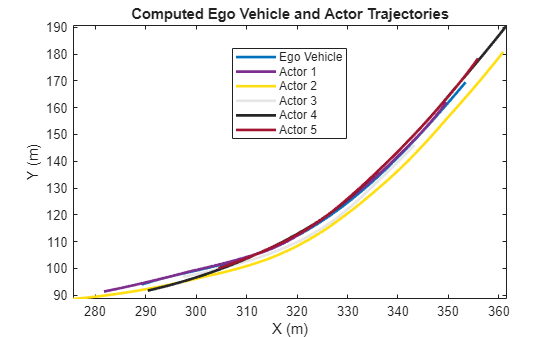```figure, plot(egoWaypoints(:,1),egoWaypoints(:,2),... Color=[0 0.447 0.741],LineWidth=2) hold on for i =1:size(actors,1) plot(nonEgoWaypoints{i}(:,1),... nonEgoWaypoints{i}(:,2),... Color=nonEgoProperties.Color(i,:),LineWidth=2) end axis("tight") xlabel("X (m)") ylabel("Y (m)") title("Computed Ego Vehicle and Actor Trajectories") legend("Ego Vehicle", "Actor 1", "Actor 2", "Actor 3",... "Actor 4","Actor 5","Location","Best") hold off```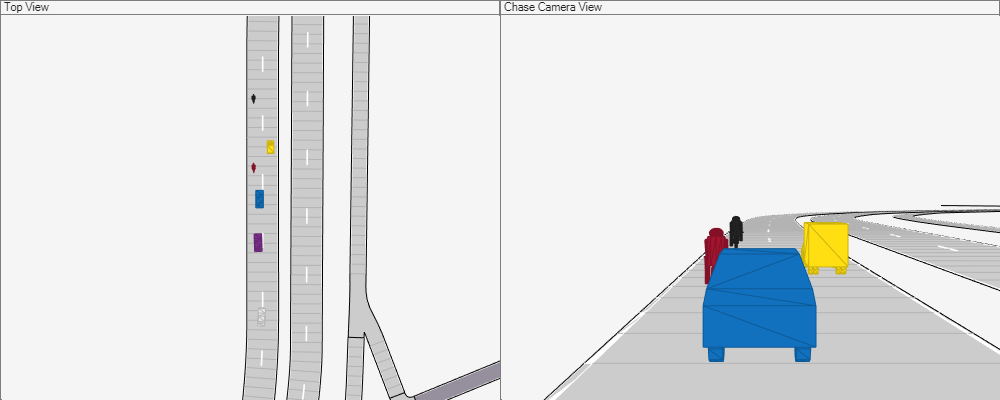### Simulate and Visualize Generated Scenario

Plot the scenario and the corresponding chase plot. Run the simulation to visualize the generated driving scenario. The ego vehicle and the non-ego actors follow their respective trajectories.

```% Create a custom figure window to show the scenario and chase plot figScene = figure(Name="Driving Scenario",... Tag="ScenarioGenerationDemoDisplay"); set(figScene,Position=[0, 0, 1000, 400]); movegui(figScene,"center"); % Add the chase plot hCarViewPanel = uipanel(figScene,... Position=[0.5 0 0.5 1],Title="Chase Camera View"); hCarPlot = axes(hCarViewPanel); chasePlot(ego,Parent=hCarPlot, Meshes="on"); % Add the top view of the generated scenario hViewPanel = uipanel(figScene,... Position=[0 0 0.5 1],Title="Top View"); hCarPlot = axes(hViewPanel); chasePlot(ego,Parent=hCarPlot,Meshes="on",... ViewHeight=120, ViewPitch=90, ViewLocation=[0, 0]); % Run the simulation while advance(scenario) pause(0.01) end```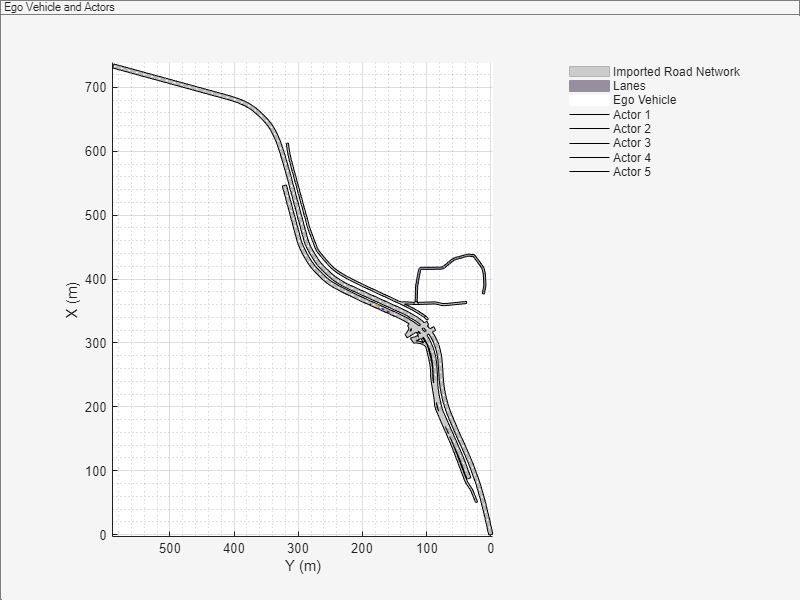### Export to ASAM OpenSCENARIO

You can also export the scenario to ASAM OpenSCENARIO file.

```warning("off","driving:scenario:ExportOpenScenarioODWarning"); export(scenario, "OpenSCENARIO", "PlaybackScenarioExample.xosc"); warning("on","driving:scenario:ExportOpenScenarioODWarning");```

The ASAM OpenSCENARIO file can be imported into other tools to visualise add use the same scenario.

### Summary

This example shows how to automatically generate a virtual driving scenario from vehicle data recorded using the GPS and lidar sensors.

### Helper Functions

helperGetEgoData

This function reads the ego vehicle data from a text file and converts into a structure.

```function [egoData] = helperGetEgoData(egoFile) %Read the ego vehicle data from text file fileID = fopen(egoFile); content = textscan(fileID,'%f %f %f'); fields = {'lat','lon','Time'}; egoData = cell2struct(content,fields,2); fclose(fileID); end```

helperGetNonEgoData

This function reads the processed lidar data and non-ego actor properties in the from text files. You can convert it into a structure. The processed lidar data contains information about the position of non-ego actors, were the non-ego actor properties contains type, dimention and colour information about respective non-ego actors.

```function [nonEgoPos, nonEgoProperties] = ... helperGetNonEgoData(nonEgoPosFile, nonEgoPropertiesFile) % Read the processed lidar data of non-ego actors from text file. fileID1 = fopen(nonEgoPosFile); content = textscan(fileID1,'%d %f %f %f %f'); newcontent{1} = content{1}; newcontent{2} = [content{2} content{3} content{4}]; newcontent{3} = content{5}; fields = {'ActorID','Position','Time'}; nonEgoPos = cell2struct(newcontent,fields,2); fclose(fileID1); fileID2 = fopen(nonEgoPropertiesFile); content = textscan(fileID2,'%d %f %f %f %f %f %f'); newcontent{1} = content{1}; newcontent{2} = content{2}; newcontent{3} = content{3}; newcontent{4} = content{4}; newcontent{5} = [content{5} content{6} content{7}]; fields = {'ClassID','Length','Width','Height','Color'}; nonEgoProperties = cell2struct(newcontent,fields,2); fclose(fileID2); end```

helperComputeEgoData

This function calculates the speed and heading angle of the ego vehicle based on the trajectory waypoints and the timestamps.

```function [egoSpeed, egoAngle] = ... helperComputeEgoData(egoData, X, Y) egoTime = egoData.Time; timeDiff = diff(egoTime); points = [X Y]; difference = diff(points, 1); distance = sqrt(sum(difference .* difference, 2)); egoSpeed = distance./timeDiff; egoAngle = atan(diff(Y)./diff(X)); egoAngle(end+1) = egoAngle(end); egoSpeed(end+1) = egoSpeed(end); end```

helperComputeNonEgoData

This function calculates the speed and heading angle of each non-ego actor based on the trajectory waypoints and timestamps. The speed and heading angle are calculated relative to the ego vehicle.

```function [nonEgoSpeed, nonEgoWaypoints] = ... helperComputeNonEgoData(... egoData, egoWaypoints, nonEgoData, egoAngle) actors = unique(nonEgoData.ActorID); numActors = size(actors,1); nonEgoWaypoints = cell(numActors, 1); nonEgoSpeed = cell(numActors, 1); for i = 1:numActors id = actors(i); idx = find([nonEgoData.ActorID] == id); actorXData = nonEgoData.Position(idx,1); actorYData = nonEgoData.Position(idx,2); actorTime = nonEgoData.Time(idx); actorWaypoints = [0 0]; % Compute the trajectory waypoints of non-ego actor [sharedTimeStamps,nonEgoIdx,egoIdx] = ... intersect(actorTime,egoData.Time,"stable"); tempX = actorXData(nonEgoIdx); tempY = actorYData(nonEgoIdx); relativeX = -tempX .* cos(egoAngle(egoIdx)) + tempY .* sin(egoAngle(egoIdx)); relativeY = -tempX .* sin(egoAngle(egoIdx)) - tempY .* cos(egoAngle(egoIdx)); actorWaypoints(nonEgoIdx,1) = egoWaypoints(egoIdx,1) + relativeX; actorWaypoints(nonEgoIdx,2) = egoWaypoints(egoIdx,2) + relativeY; % Compute the speed values of non-ego actor timeDiff = diff(sharedTimeStamps); difference = diff(actorWaypoints, 1); distance = sqrt(sum(difference .* difference, 2)); actorSpeed = distance./timeDiff; actorSpeed(end+1) = actorSpeed(end); % Smooth the trajectory waypoints of non-ego actor actorWaypoints = smoothdata(actorWaypoints,"sgolay"); % Store the values of trajectory waypoints and speed computed of each non-ego actor nonEgoWaypoints(i) = {actorWaypoints}; nonEgoSpeed(i) = {actorSpeed'}; end end```